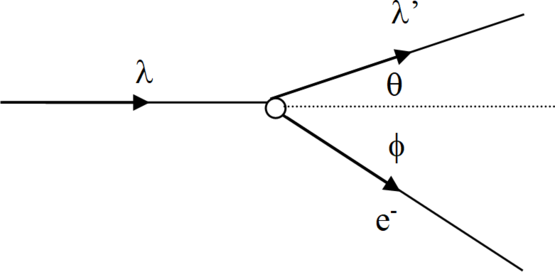$$\require{cancel}$$

# 4.2: Compton Scattering

•• Contributed by Paul D'Alessandris
• Professor (Engineering Science and Physics) at Monroe Community College

Compton scattering refers to the scattering of light off of free electrons. Experimentally, it’s impossible to create a target of completely free electrons. However, if the incident photons have energy much greater than the typical binding energies of electrons to atoms, the electrons will be “knocked off” of the atoms by the photons and act as free particles. Therefore, Compton scattering typically refers to scattering of high energy photons off of atomic targets.

If light was purely a wave phenomenon, an incoming wave with a specific frequency would cause the electron to oscillate with the same frequency. The oscillating electron would then emit electromagnetic waves of this frequency. Thus, the scattered light and incoming light would have, to within a slight variation due to the Doppler effect for light, the same frequency. This is not what is seen experimentally.

Instead, let’s imagine light to be a stream of photons and analyze the collision of a photon and an electron by energy and momentum conservation. Consider an incident photon of wavelength $$\lambda$$ striking a stationary electron. The photon scatters to angle $$\theta'$$ (and new wavelength $$\lambda'$$) and the electron to angle $$\phi$$.To analyze, apply energy conservation:

$E + mc^2 = E' + E_e$

$$x$$-momentum conservation:

$pc + 0 = p'c \cos \theta + p_e c \cos \phi$

and $$y$$-momentum conservation:

$0 + 0 = p'c \sin \theta + p_e c \sin \phi$

For photons, $$E = pc$$, so the momentum equations can be written as:

$p_x: E = E' \cos \theta + p_e \cos \phi$

$p_x: 0 = E' \cos \theta - p_e \sin \phi$

Experimentally, it’s easier to detect the scattered photon than the scattered electron, so we’ll eliminate the electron parameters and derive an interrelationship between the various photon parameters. To eliminate $$\phi$$, solve $$x$$-momentum and $$y$$-momentum for $$\cos \phi$$ and $$\sin \phi$$, and then square and add them together:

Solve the energy conservation equation for $$E_e$$:

$E_e = E + mc^2 - E'$

$E_e^2 = ( E + mc^2 -E')^2 \label{eq20}$

Plug the two previous results into Equation \ref{eq20} to eliminate the electron variables:

$E_e^2 = (p_ec)^2 + (mc^2)^2$

$( E + mc^2 -E')^2 = E^2 - 2EE' \cos \theta E'^2 + (mc^2)^2$

Six terms cancel and the equation greatly simplifies

$2Emc^2 - 2E'mc^2 - 2EE' = -2EE' \cos \theta$

Rearranging yields

This result directly relates the incoming wavelength to the scattered wavelength and the scattering angle. All of these parameters are easily measured experimentally. For his theoretical explanation and experimental verification of high energy photon scattering, the American Arthur Compton was awarded the Nobel Prize in 1927.

# Using the Compton Scattering Relationship

An 800 keV photon collides with an electron at rest. After the collision, the photon is detected with 650 keV of energy. Find the kinetic energy and angle of the scattered electron.

The fundamental relationship for Compton scattering is

$\lambda' - \lambda = \dfrac{hc}{mc^2}( 1- \cos \theta)$

where

• $$\lambda'$$ is the scattered photon wavelength,
• $$\lambda$$ is the incident photon wavelength,
• and $$\theta$$ is the angle of the scattered photon.

To find the kinetic energy of the scattered electron does not require using the Compton formula. If the photon initially has $$800\, \text{keV}$$, and after scattering has $$650 \text{keV}$$, then $$150, \text{keV}$$ must have been transferred to the electron. Thus, $$KE_{electron} = 150 \text{keV}$$.

Finding the angle of the scattered electron does involve the Compton relation. First, convert the photon energies into wavelengths:

\begin{align} E_{photon} &= \frac{hc}{\lambda} \\[5pt] \lambda &= \frac{hc}{E} \\[5pt] &= \frac{1240 \text{ eVnm}}{800 \text{ keV}} = 1.55 \times 10^{-3} \text{ nm} \\[5pt] & = \frac{1240 \text{ eVnm}}{650 \text{ keV}} = 1.91 \times 10^{-3} \text{ nm} \end{align}

then use the relationship

$\lambda' - \lambda = \frac{hc}{mc^2}(1-\cos\theta)$

$1.91\times 10^{-3} - 1.55 \times 10^{-3} = \lambda = \frac{1240 \text{ eVnm}}{511 \text{ keV}}(1-\cos\theta)$

$0.1484 = (1-\cos\theta)$

$\theta = 31.6°$

However, this is the scattering angle of the photon, not the electron!

To find the electron’s scattering angle, apply momentum conservation in the direction perpendicular to the initial photon direction.

$0 = p_{\text{scattered photon}}c(\sin\theta)-p_{electron}c(\sin\phi)$

$p_{\text{scattered photon}}c(\sin\theta) =p_{electron}c(\sin\phi)$

$E_{\text{scattered photon}}c(\sin\theta) = \sqrt{E_{electron}^2-(mc^2)^2}(\sin\theta)$

$650\sin(31.6)=\sqrt{(511+150)^2-(511)^2}(\sin\phi)$

$\sin\phi =0.813$

$\phi = 54.4$